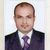# QlikView App Dev

Discussion Board for collaboration related to QlikView App Development.

Announcements
Now Live: Qlik Sense SaaS Simplified Authoring – Analytics Creation for Everyone: READ DETAILS
cancel
Showing results for
Did you mean:Master

## Need Week count

Hi all,

I want to calculate the count of  items   of current week  dynamically   how to write the exp?

I have a weeknumber column in data

=count(Item)

1 Solution

Accepted SolutionsMVP

Your expression seems ok and working fine try some of this

=count( {<Flag={1},WeekNumber = {" \$(=max(WeekNumber))"}>} Item)

Or

=count( {<Flag={1},WeekNumber = {" \$(=max(WeekNumber))"}>} [Item])

28 RepliesSpecialist III

count({\$<weeknumber={'\$(=max(weeknumber))'}>} Item)Not applicable

Try this

Count({<weeknumber={\$(=max(weeknumber))}>}Item)MVP

If your weeknumber field has values like, 1,2,... try like:

=Count({<weeknumber={'\$(=Week(Today()))'}>}Item)Partner - Creator III

=count(distinct if(weekweeknumber)=  week(today()),id_produs))MVP

Try with this expression

=count( {<weeknumber = {" \$(=max( weeknumber  ))"}>} Item)Master
Author

but,

in my data  weeknumber based on data     I need max of week   based on data     [I don't need based on calendar week]

for example:    in my data  maxweek is   30       so I need to show count of item of 30th week

weeknumber            ---- this is not calendar week

30

29MVP

Let me know the field weeknumber is string field if not by this statement =max( weeknumber  ) you get Max weeknumber that is 30 if they are number.Master
Author

yes,its number Anand,

in text object    =max(weeknumber) showing 30.

in chart expression  I have like this

Count({<Flag={1}>} Item)

I tried below

=count( {<Flag={1},WeekNumber = {" \$(=max(WeekNumber))"}>} Item])   its not workingMVP

May be you need to remove the additional square bracket around Item?

=Count({<Flag = {1}, WeekNumber = {" \$(=max(WeekNumber))"}>} Item])

So, use it like this

=Count({<Flag = {1}, WeekNumber = {" \$(=max(WeekNumber))"}>} Item)